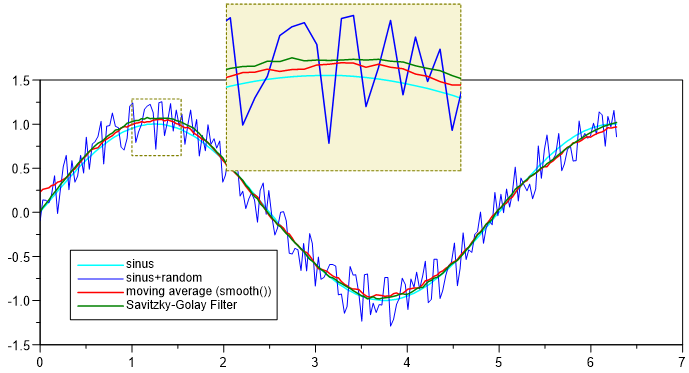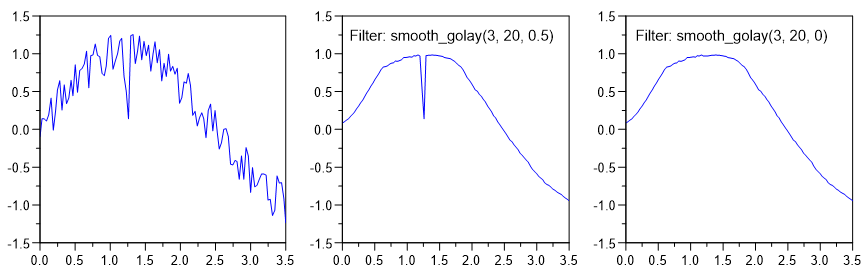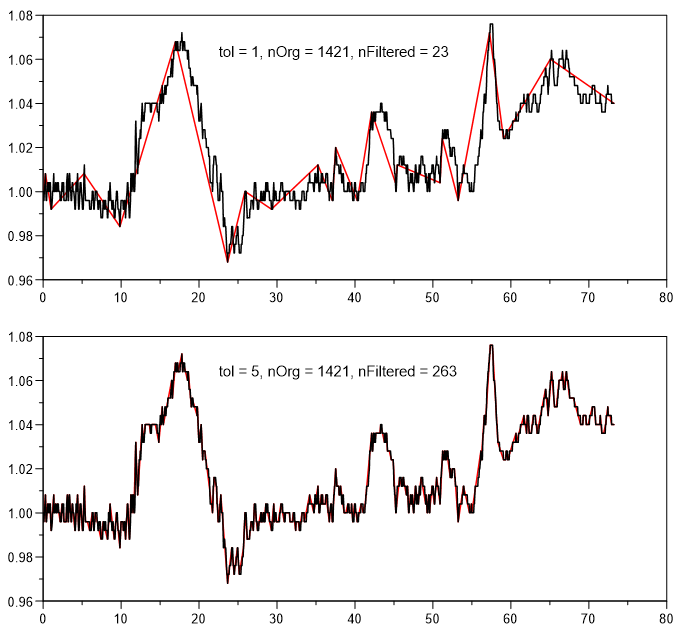# Filter Functions for XY datasets¶

Filter functions are new with UniPlot 4.0. They offer more possibilities to filter and transform the original data of a dataset. A filter, for example, can calculate a cubic spline or remove unwanted points from a curve. They are stored inside the dataset. Filter functions are recalculated when the original data is edited or the datasource of a dataset has changed. The filter functions can be specified for XY datasets (1D or 2D). When a dataset is plotted, the result of the filter function is displayed in the diagram. Optionally, the original data can be plotted as symbols.

For each dataset a list of filter functions can be specified. The first function will use the original data as its input. All other functions will use the output of the previous filter function.

The results of the filter functions are saved in the dataset. If you pass a UniPlot document with filtered datasets to another UniPlot user the result will be displayed correctly, but recalculation of the filter function is only possible if the filter function used in the document are available on the computer.

Filter functions are UniScript functions which can be defined by the user. The following functions can be used as a template for your own filter functions. For more information on writing filter function, move to the bottom of this help topic.

## List of filter functions¶

The source code of the following functions can be found in `script/rs_xy_filt_funcs.ic`.

### abs¶

```abs()
```

abs() returns the absolute value.

### bit¶

Extracts a bit form a vector. iBit in the range 1 to 64. The data can be scaled.

```bit(iBit, rsScaleFactor, rsScaleOffset)
```

iBit: Range 1 to 64

If rsScaleFactor is set to 1 and rsScaleOffset is set to 0, the result is in the range 0 to 1.

### min¶

```min()
```

Calulates the minimum of all given y values.

### max¶

```max()
```

Calulates the maximum of all given y values.

### mean¶

```mean()
```

Calulates the mean value of all given y values.

### median¶

```median(nPercent)
```

Calculates the median of all given y values. Sorts the data in ascending order and picking the middle one if nPercent == 50 percent. The index of the data point can be specified in percent of the number of data points.

### smooth¶

```smooth()
smooth(nNeighbor)
```

Smooth a dataset with the moving average.

The optional parameter `nNeighbor` specifies the number of points used the moving average. nNeighbor specifies the window width. The window width is `2 * nNeighbor + 1`.

The If the parameter is not specified, it is set to 10.

With the help of the optional parameter `PeakFilterFactor` peaks can be keept in the filtered signal. If set to 0 all peaks are smoothed.

This is how the Peak-Filter works:

• The smooth function will be applied on the original data.
• The difference between the original and the filtered data is calculated.
• The difference is multiplied with PeakFilterFactor (Range 0 to 1) = dy.
• All points of the original data with a difference greater than dy are inserted into the filtered signal.

### smooth_median¶

```smooth_median(nNeighbor)
```

Running median. nNeighbor is the window size of the moving window in the range 3 to 1025. Even numbers will be rounded up to the next odd number. For each window the middle value found is used. The filter can be used to reduce random noise and spikes. (see moving_median).

Example: `smooth_median(5)`

5 consecutive points are sorted ascending and the third point is used. For a window size of 5, the first 2 point and the last 2 points are not altered.

given: `[5, 7, 6, 4, 27, 8, 4, 5]`

result: `[5, 7, 6, 7, 6,  8, 4, 5]`

Example:### smooth_golay (Savitzky-Golay)¶

```smooth_golay(nPolynomialOrder, nWindowSize)
smooth_golay(nPolynomialOrder, nWindowSize, PeakFilterFactor)
```

A better procedure than simply averaging points is to perform a least squares fit of a small set of consecutive data points to a polynomial and take the calculated central point of the fitted polynomial curve as the new smoothed data point.

The smoothing effect of the Savitzky-Golay algorithm is not so aggressive as in the case of the moving average and the loss and/or distortion of vital information is comparatively limited.Peak-Filter. The method is identical to the smooth-funcition.### spline¶

```spline()
spline(nPoints)
```

Calculates a cubic spline that will cross all datapoints.

nPoints: Number of spline points (Default = 1000). The number must be in the range of 10 to 100000.

### fitspline¶

```fit_spline()
fit_spline(smoothfactor)
fit_spline(smoothfactor, nPoints)
```

The Fit Spline function fits a smoothing spline using a smoothing parameter you specify.

smoothfactor: A value of 0 meas no smoothing (i.e. the spline will cross all original data points) and a value greater as 0 will create a smooting spline.

nPoints: Number of points in the range 10 to 100000.

### akimaspline¶

```akimaspline()
akimaspline(nPoints)
```

Akima spline interpolation better copes with discontinuities in a time series than normal cubic splines. The interpolation approximates a manually drawn curve better than the ordinary splines, but the second derivation is not continuous.

nPoints: Number of spline points in the range 10 to 100000.

### polyfit¶

```polyfit()
polyfit(polynomOrder)
polyfit(polynomOrder, nPoints, nInfoText)
polyfit(polynomOrder, nPoints, nInfoText, xMin, xMax)
```

Polynomial curve fitting.

polyfit finds the coefficients of a polynomial p(x) of degree n that fits the data, p(x(i)) to y(i), in a least squares sense. Creates a fit polynom of degree 0 to 9. The polynomial of degree 1 is the regression line.

polynomOrder: Order of polynom (default = 1). 1 creates a straight line (linear regression).

nPoints: Number of points of the fitting curve (default = 1000). The number of points should be in the range 2 to 100000.

nInfoText: 0 = no text, 1 = Polynom parameter, 2 = Polynom parameter and R2 (coefficient of determination).

xMin: Start value, can be smaller than the first x-data value. If set to 1e10, the x-minimum of the dataset will be used.

xMax: End value, can be larger than the last x-data value. If set to 1e10, the x-maximum of the dataset will be used.

### interpol¶

```interpol()
interpol(nPoints)
```

Calculates extra points for a curve through linear interpolation.

nPoints: Number of points of the new curve or a list of x-coordinates separated by a comma.

### interpol2¶

```interpol2(xmin, xmax, xdelta)
```

Calculates extra points for a curve from xmin to xmax with an increment of xdelta through linear interpolation.

The dataset must be monoton. If xmin or xmax is out of x-range the range will be corrected. The function cannot perform an extrapolation.

### sort¶

```sort()
```

Sorts the data in ascending order based on the x coordinate.

### simplify¶

```simplify()
simplify(nTolerance)
```

Polyline simplification using the Douglas-Peucker algorithm.

nTolerance: Specified the maximum distance between the original curve and the simplified curve. A value of 1 sets a tolerance of 10%. The values 2,3,4,5, etc. always divide the tolerance by 2. Therfore a value of 4 is a tolerance of 1.25%. A value of 30 sets a tolerance of almost 0% Default value is 5.### boundary¶

```boundary()
boundary(type)
boundary(type, width)
```

Calculates the upper, lowert or upper and lower hull curve.

type: 0 - upper hull (Default value = 0).

type: 1 - lower hull.

type: 2 - upper and lower hull.

width: Class width in percent (Default value = 5%).

### step¶

```step()
step(nType)
```

Calculates a cityscape or skyline curve

nType: 0 - Curve starts horizontal.

nType: 1 - Curve starts vertical (Default value = 1).

### integrate¶

```integrate()
```

Calculates the integral curve using the trapezoidal method.

### integrate_cycle¶

```integrate_cycle(xCycleLength, xCycleStart, nCycle)
```

Calculates for each cycle the integral curve using the trapezoidal method.

xCycleLength: Length of a cycle in x-coordinates, example: 0.2s or 720 °CA.

xCycleStart: First Point in x-coordinates, example 0s or -360 °CA. xCycleStart is set to x if less than x.

nCycle: Number of Cycles. 0 for all cycles.

### cycle_value¶

```cycle_value(nType, xCycleLength, xCycleStart, nCycle)
```

Calculates one of the following values for each cycle:

nType: 1: Mean Value, 2: Max Value, 3: Min Value

xCycleLength: Length of a cycle in x-coordinates, example: 0.2s or 720 °CA.

xCycleStart: First Point in x-coordinates, example 0s or -360 °CA. xCycleStart is set to x if less than x.

nCycle: Number of Cycles. 0 for all cycles.

### differentiate¶

```differentiate()
```

Calculates the derivative.

### extract¶

```extract(xmin, xmax)
```

Extracts data from a curve in the range xmin to xmax. The points at the lower and upper limit are calculated by linear interpolation.

The x-coordinates must be strictly increasing.

see extract_points

### extract_points¶

```extract_points(imin, imax)
```

The extract_points function extracts the points from imin to imax.

imin: First point (imin >= 1 and <= Number of points - 2).

imax: Last point. If imax is negative the points are counted starting from the end. Example for a dataset with 17 points:

extract_points(1,22) equivalent to extract_points(1,17)

extract_points(1,-1) equivalent to extract_points(1,17)

extract_points(1,0) equivalent to extract_points(1,17)

extract_points(3,-3) equivalent to extract_points(3,15)

### find¶

```find(min, max, Axis, Type)
```

Returns data points in the range min to max. If no data point is found the functions returns the value 0.

Axis is one of the following values:

1: Search x-coordinates

2: Search y-coordinates

Type is one of the followin values:

0: Absolute values (no scaling)

1: Relative values: Values are divided by the maximum before the search (xy / xymax).

2: Relative values: Valurs are scaled to the range 0 to 1: (xy - xymin) / (xymax-xymin).

If Type is 1 or 2 min and max should be set in the range 0 to 1

Example: To find all y-data points in the range 90% of the maximum value, the filter string would be find(0.9, 1.0, 2, 1).

### remove_duplicate_points¶

```remove_duplicate_points()
```

Removes all duplicate consecutive datapoints with equal x coordinates except the first data point.

### correction_factor¶

```correction_factor(x,y)
```

Corrects the y-coordinate using a variable correction factor.

## Writing Filter Functions¶

Below is an example on how to write filter functions

```def _xy_filter_func_scale(hData, a, b)
{
if (nargsin() == 1) {
_a = 1;
_b = 0;
} else if (nargsin() == 2) {
_a = a;
_b = 0;
} else if (nargsin() == 3) {
_a = a;
_b = b;
}
xy = XYGetData(hData);
x = xy[;1];
y = xy[;2];
y = _a * y + _b;
return XYSetData(hData, x, y, TRUE);  // Don't forget the TRUE!
}
def _xy_filter_info_scale()
{
svInfo = ["Y-Skalierung";
"scale(a,b)\n\nScales the y-coordinates with the help" + ....
"of the equation y = a*y + b";
"";
"2";
"Factor a:1:-1e9:1e9";
"Offset b:0:-1e9:1e9"];
return svInfo;
}
```

To define a new filter function, two functions need to be written. One function calculates the data, the other provides information to the user interface. In the example above these two functions are `_xy_filter_func_scale` and `_xy_filter_info_scale`.

The filter function names begin with `_xy_filter_func_`, followed by the functions user namen in this case `scale`.

The functions can be saved in a file with en extension `.ic` in UniPlot’s autoload directory. When UniPlot is started the functions will be loaded automatically.

The info function returns a string vector with at least 4 elements:

Element Meaning
svInfo Function name.
svInfo Info text shown in the Help windows. If the text is long it can be spaced over various lines as in the example. The rows will be linked with `+ ....`.
svInfo Not used. Must be an empty string (“”).
svInfo Number of parameters “0” to “8”
svInfo[5,6,..] (Optional) Parameter name, default value and range separated by a colon (:).

The func function makes the calculation. The dataset handle will always be the first parameter. Data from the dataset can be accessed using the handle (XYGetData). The results will be written with the XYSetData function. The other parameters are option and must match the definition in the info function.

History

Version Description
R2022.2 correction_factor

id-1866448